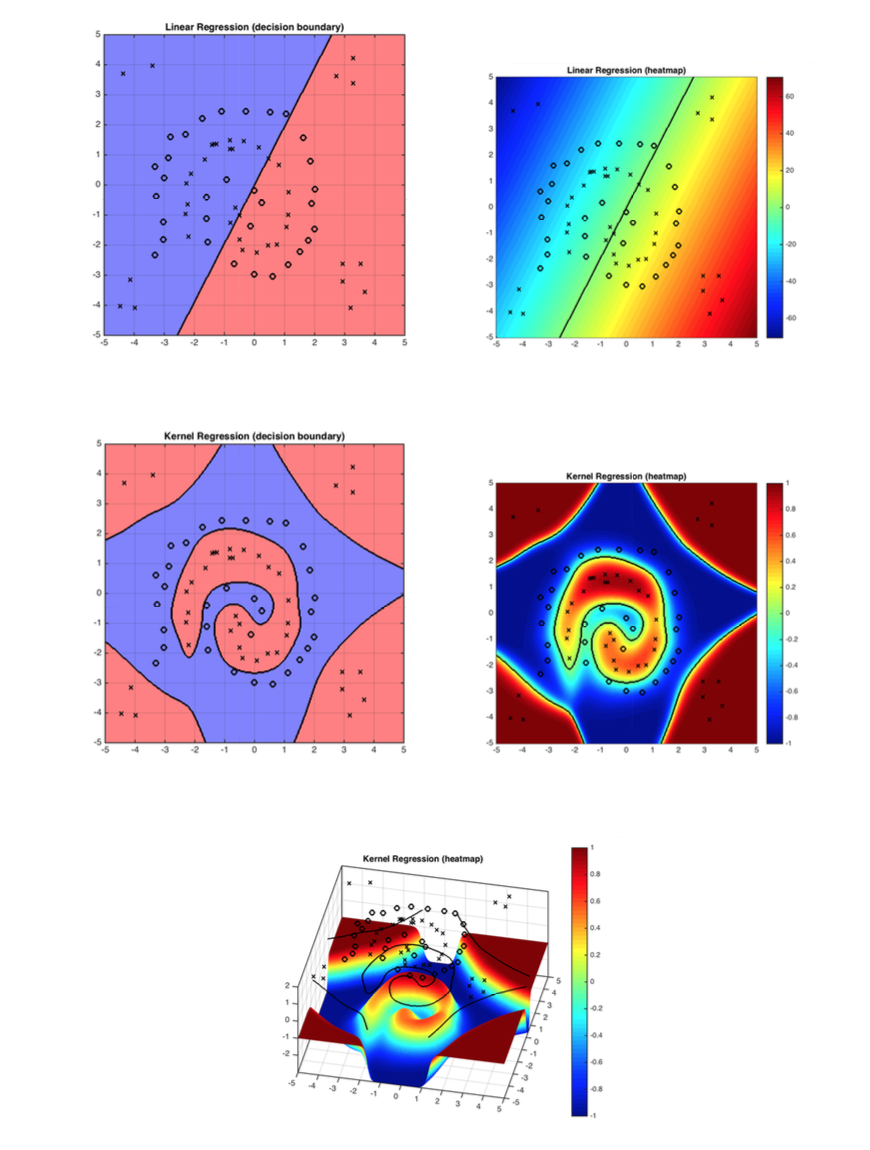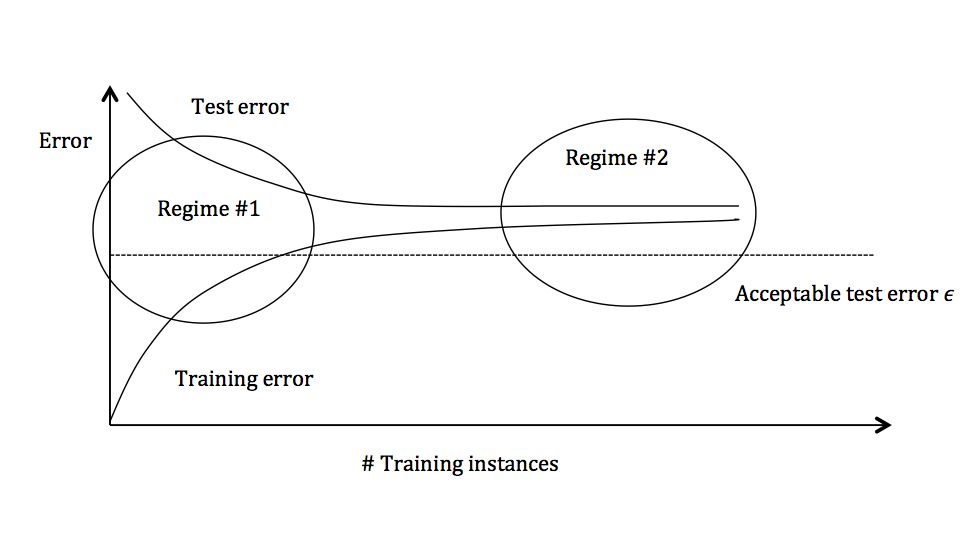Lecture 13: Kernels

Video II

Linear classifiers are great, but what if there exists no linear decision boundary? As it turns out, there is an elegant way to incorporate non-linearities into most linear classifiers.

Handcrafted Feature Expansion

We can make linear classifiers non-linear by applying basis function (feature transformations) on the input feature vectors. Formally, for a data vector $\mathbf{x}\in\mathbb{R}^d$, we apply the transformation $\mathbf{x} \rightarrow \phi(\mathbf{x})$ where $\phi(\mathbf{x})\in\mathbb{R}^D$. Usually $D \gg d$ because we add dimensions that capture non-linear interactions among the original features.

Advantage: It is simple, and your problem stays convex and well behaved. (i.e. you can still use your original gradient descent code, just with the higher dimensional representation)

Disadvantage: $\phi(\mathbf{x})$ might be very high dimensional.

Consider the following example: $\mathbf{x}=\begin{pmatrix}x_1\\ x_2\\ \vdots \\ x_d \end{pmatrix}$, and define $\phi(\mathbf{x})=\begin{pmatrix}1\\ x_1\\ \vdots \\x_d \\ x_1x_2 \\ \vdots \\ x_{d-1}x_d\\ \vdots \\x_1x_2\cdots x_d \end{pmatrix}$.

Quiz: What is the dimensionality of $\phi(\mathbf{x})$?

This new representation, $\phi(\mathbf{x})$, is very expressive and allows for complicated non-linear decision boundaries - but the dimensionality is extremely high. This makes our algorithm unbearable (and quickly prohibitively) slow.

The Kernel Trick

The kernel trick is a way to get around this dilemma by learning a function in the much higher dimensional space, without ever computing a single vector $\phi(\mathbf{x})$ or ever computing the full vector $\mathbf{w}$. It is a little magical.

It is based on the following observation: If we use gradient descent with any one of our standard loss functions, the gradient is a linear combination of the input samples. For example, let us take a look at the squared loss:

\begin{equation} \ell(\mathbf{w}) = \sum_{i=1}^n (\mathbf{w}^\top \mathbf{x}_i-y_i)^2\label{eq:c15:sql} \end{equation} The gradient descent rule, with step-size/learning-rate $s>0$ (we denoted this as $\alpha>0$ in our previous lectures), updates $\mathbf{w}$ over time, \begin{equation} w_{t+1} \leftarrow w_t - s(\frac{\partial \ell}{\partial \mathbf{w}})\ \textrm{ where: } \frac{\partial \ell}{\partial \mathbf{w}}=\sum_{i=1}^n \underbrace{2(\mathbf{w}^\top \mathbf{x}_i-y_i)}_{\gamma_i\ :\ \textrm{function of $\mathbf{x}_i, y_i$}} \mathbf{x}_i = \sum_{i=1}^n\gamma_i \mathbf{x}_i \end{equation} We will now show that we can express $\mathbf{w}$ as a linear combination of all input vectors, \begin{equation} \mathbf{w}=\sum_{i=1}^n \alpha_i {\mathbf{x}}_i.\label{eq:c15:alphas} \end{equation} Since the loss is convex, the final solution is independent of the initialization, and we can initialize $\mathbf{w}^0$ to be whatever we want. For convenience, let us pick $\mathbf{w}_0=\begin{pmatrix}0 \\ \vdots \\ 0\end{pmatrix}$. For this initial choice of $\mathbf{w}_0$, the linear combination in $\mathbf{w}=\sum_{i=1}^n \alpha_i {\mathbf{x}}_i$ is trivially $\alpha_1=\dots=\alpha_n=0$. We now show that throughout the entire gradient descent optimization such coefficients $\alpha_1,\dots,\alpha_n$ must always exist, as we can re-write the gradient updates entirely in terms of updating the $\alpha_i$ coefficients: \begin{align} \mathbf{w}_1=&\mathbf{w}_0-s\sum_{i=1}^n2(\mathbf{w}_0^\top \mathbf{x}_i-y_i)\mathbf{x}_i=\sum_{i=1}^n \alpha_i^0 {\mathbf{x}}_i-s\sum_{i=1}^n\gamma_i^0\mathbf{x}_i=\sum_{i=1}^n\alpha_i^1\mathbf{x}_i&(\textrm{with $\alpha_i^1=\alpha_i^0-s\gamma_i^0$})\nonumber\\ \mathbf{w}_2=&\mathbf{w}_1-s\sum_{i=1}^n2(\mathbf{w}_1^\top \mathbf{x}_i-y_i)\mathbf{x}_i=\sum_{i=1}^n \alpha_i^1\mathbf{x}_i-s\sum_{i=1}^n\gamma_i^1\mathbf{x}_i=\sum_{i=1}^n\alpha_i^2\mathbf{x}_i&(\textrm{with $\alpha_i^2=\alpha_i^1\mathbf{x}_i-s\gamma_i^1$})\nonumber\\ \mathbf{w}_3=&\mathbf{w}_2-s\sum_{i=1}^n2(\mathbf{w}_2^\top \mathbf{x}_i-y_i)\mathbf{x}_i=\sum_{i=1}^n \alpha_i^2\mathbf{x}_i-s\sum_{i=1}^n\gamma_i^2\mathbf{x}_i=\sum_{i=1}^n\alpha_i^3\mathbf{x}_i&(\textrm{with $\alpha_i^3=\alpha_i^2-s\gamma_i^2$})\nonumber\\ \cdots & \qquad\qquad\qquad\cdots &\cdots\nonumber\\ \mathbf{w}_t=&\mathbf{w}_{t-1}-s\sum_{i=1}^n2(\mathbf{w}_{t-1}^\top \mathbf{x}_i-y_i)\mathbf{x}_i=\sum_{i=1}^n \alpha_i^{t-1}\mathbf{x}_i-s\sum_{i=1}^n\gamma_i^{t-1}\mathbf{x}_i=\sum_{i=1}^n\alpha_i^t\mathbf{x}_i&(\textrm{with $\alpha_i^t=\alpha_i^{t-1}-s\gamma_i^{t-1}$})\nonumber \end{align}

Formally, the argument is by induction. $\mathbf{w}$ is trivially a linear combination of our training vectors for $\mathbf{w}_0$ (base case). If we apply the inductive hypothesis for $\mathbf{w}_t$ it follows for $\mathbf{w}_{t+1}$.

The update-rule for $\alpha_i^t$ is thus \begin{equation} \alpha_i^t=\alpha_i^{t-1}-s\gamma_i^{t-1}, \textrm{ and we have } \alpha_i^t=-s\sum_{r=0}^{t-1}\gamma_i^{r}. \end{equation} In other words, we can perform the entire gradient descent update rule without ever expressing $\mathbf{w}$ explicitly. We just keep track of the $n$ coefficients $\alpha_1,\dots,\alpha_n$. Now that $\mathbf{w}$ can be written as a linear combination of the training set, we can also express the inner-product of $\mathbf{w}$ with any input ${\mathbf{x}}_i$ purely in terms of inner-products between training inputs: \begin{equation} \mathbf{w}^\top {\mathbf{x}}_j=\sum_{i=1}^n \alpha_i {\mathbf{x}}_i^\top{\mathbf{x}}_j.\nonumber \end{equation} Consequently, we can also re-write the squared-loss from $\ell(\mathbf{w}) = \sum_{i=1}^n (\mathbf{w}^\top \mathbf{x}_i-y_i)^2$ entirely in terms of inner-product between training inputs: \begin{equation} \ell(\mathbf{\alpha}) = \sum_{i=1}^n \left(\sum_{j=1}^n\alpha_j\mathbf{x}_j^\top \mathbf{x}_i-y_i\right)^2\label{eq:c15:sql:ip} \end{equation} During test-time we also only need these coefficients to make a prediction on a test-input $x_t$, and can write the entire classifier in terms of inner-products between the test point and training points: \begin{equation} h({\mathbf{x}}_t)=\mathbf{w}^\top {\mathbf{x}}_t=\sum_{j=1}^n\alpha_j{\mathbf{x}}_j^\top {\mathbf{x}}_t. \end{equation} Do you notice a theme? The only information we ever need in order to learn a hyper-plane classifier with the squared-loss is inner-products between all pairs of data vectors.

Inner-Product Computation

Let's go back to the previous example, $\phi(\mathbf{x})=\begin{pmatrix}1\\ x_1\\ \vdots \\x_d \\ x_1x_2 \\ \vdots \\ x_{d-1}x_d\\ \vdots \\x_1x_2\cdots x_d \end{pmatrix}$.

The inner product $\phi(\mathbf{x})^\top \phi(\mathbf{z})$ can be formulated as: \begin{equation} \phi(\mathbf{x})^\top \phi(\mathbf{z})=1\cdot 1+x_1z_1+x_2z_2+\cdots +x_1x_2z_1z_2+ \cdots +x_1\cdots x_dz_1\cdots z_d=\prod_{k=1}^d(1+x_kz_k)\text{.}\label{eq:c15:poly} \end{equation} The sum of $2^d$ terms becomes the product of $d$ terms. We can compute the inner-product from the above formula in time $O(d)$ instead of $O(2^d)$! We define the function \begin{equation} \underbrace{\mathsf{k}(\mathbf{x}_i,\mathbf{x}_j)}_{\text{this is called the} \textbf{ kernel function}}=\phi(\mathbf{x}_i)^\top \phi(\mathbf{x}_j). \end{equation} With a finite training set of $n$ samples, inner products are often pre-computed and stored in a Kernel Matrix: \begin{equation} \mathsf{K}_{ij}=\phi(\mathbf{x}_i)^\top \phi(\mathbf{x}_j). \end{equation} If we store the matrix $\mathsf{K}$, we only need to do simple inner-product look-ups and low-dimensional computations throughout the gradient descent algorithm. The final classifier becomes: \begin{equation} h(\mathbf{x}_t)=\sum_{j=1}^n\alpha_j\mathsf{k}(\mathbf{x}_j,\mathbf{x}_t). \end{equation}

During training in the new high dimensional space of $\phi(\mathbf{x})$ we want to compute $\gamma_i$ through kernels, without ever computing any $\phi(\mathbf{x}_i)$ or even $\mathbf{w}$. We previously established that $\mathbf{w}=\sum_{j=1}^n\alpha_j \phi(\mathbf{x}_j)$, and $\gamma_i=2(\mathbf{w}^\top \phi(\mathbf{x}_i)-y_i)$. It follows that $\gamma_i=2(\sum_{j=1}^n \alpha_jK_{ij})-y_i)$. The gradient update in iteration $t+1$ becomes $$\alpha_i^{t+1}\leftarrow \alpha_i^t-2s(\sum_{j=1}^n \alpha_j^tK_{ij})-y_i).$$ As we have $n$ such updates to do, the amount of work per gradient update in the transformed space is $O(n^2)$ --- far better than $O(2^d)$.

General Kernels

Below are some popular kernel functions:

Linear: $\mathsf{K}(\mathbf{x},\mathbf{z})=\mathbf{x}^\top \mathbf{z}$.

(The linear kernel is equivalent to just using a good old linear classifier - but it can be faster to use a kernel matrix if the dimensionality $d$ of the data is high.)

Polynomial: $\mathsf{K}(\mathbf{x},\mathbf{z})=(1+\mathbf{x}^\top \mathbf{z})^d$.

Radial Basis Function (RBF) (aka Gaussian Kernel): $\mathsf{K}(\mathbf{x},\mathbf{z})= e^\frac{-\|\mathbf{x}-\mathbf{z}\|^2}{\sigma^2}$.

The RBF kernel is the most popular Kernel! It is a Universal approximator!! Its corresponding feature vector is infinite dimensional and cannot be computed. However, very effective low dimensional approximations exist (see this paper).

Exponential Kernel: $\mathsf{K}(\mathbf{x},\mathbf{z})= e^\frac{-\| \mathbf{x}-\mathbf{z}\|}{2\sigma^2}$

Laplacian Kernel: $\mathsf{K}(\mathbf{x},\mathbf{z})= e^\frac{-| \mathbf{x}-\mathbf{z}|}{\sigma}$

Sigmoid Kernel: $\mathsf{K}(\mathbf{x},\mathbf{z})=\tanh(\mathbf{a}\mathbf{x}^\top + c)$

Kernel functions

Can any function $\mathsf{K}(\cdot,\cdot)\rightarrow{\mathcal{R}}$ be used as a kernel?

No, the matrix $\mathsf{K}(\mathbf{x}_i,\mathbf{x}_j)$ has to correspond to real inner-products after some transformation ${\mathbf{x}}\rightarrow \phi({\mathbf{x}})$. This is the case if and only if $\mathsf{K}$ is positive semi-definite.

Definition: A matrix $A\in \mathbb{R}^{n\times n}$ is positive semi-definite iff $\forall \mathbf{q}\in\mathbb{R}^n$, $\mathbf{q}^\top A\mathbf{q}\geq 0$.

Remember $\mathsf{K}_{ij}=\phi(\mathbf{x}_i)^\top \phi(\mathbf{x}_j)$. So $\mathsf{K}=\Phi^\top\Phi$, where $\Phi=[\phi(\mathbf{x}_1),\dots,\phi(\mathbf{x}_n)]$. It follows that $\mathsf{K}$ is p.s.d., because $\mathbf{q}^\top\mathsf{K}\mathbf{q}=(\Phi^\top \mathbf{q})^2\geq 0$. Inversely, if any matrix $\mathbf{A}$ is p.s.d., it can be decomposed as $A=\Phi^\top\Phi$ for some realization of $\Phi$.

You can even define kernels over sets, strings, graphs and molecules.Figure 1: The demo shows how kernel function solves the problem linear classifiers can not solve. RBF works well with the decision boundary in this case.

Detecting High Bias and High Variance

If a classifier is under-performing (e.g. if the test or training error is too high), there are several ways to improve performance. To find out which of these many techniques is the right one for the situation, the first step is to determine the root of the problem.Figure 3: Test and training error as the number of training instances increases.

The graph above plots the training error and the test error and can be divided into two overarching regimes. In the first regime (on the left side of the graph), training error is below the desired error threshold (denoted by $\epsilon$), but test error is significantly higher. In the second regime (on the right side of the graph), test error is remarkably close to training error, but both are above the desired tolerance of $\epsilon$.

Regime 1 (High Variance)

In the first regime, the cause of the poor performance is high variance.

Symptoms:

1. Training error is much lower than test error
2. Training error is lower than $\epsilon$
3. Test error is above $\epsilon$

Remedies:

• Reduce model complexity -- complex models are prone to high variance
• Bagging (will be covered later in the course)

Regime 2 (High Bias)

Unlike the first regime, the second regime indicates high bias: the model being used is not robust enough to produce an accurate prediction.

Symptoms:

1. Training error is higher than $\epsilon$

Remedies:

• Use more complex model (e.g. kernelize, use non-linear models)